# How to flip data in Excel: reverse columns vertically and rows horizontally

The tutorial shows a few quick ways to flip tables in Excel vertically and horizontally preserving the original formatting and formulas.

Flipping data in Excel sounds like a trivial one-click task, but surprisingly there is no such built-in option. In situations when you need to reverse the data order in a column arranged alphabetically or from smallest to largest, you can obviously use the Excel Sort feature. But how do you flip a column with unsorted data? Or, how do you reverse the order of data in a table horizontally in rows? You will get all answers in a moment.

## Flip data in Excel vertically

With just a little creativity, you can work out a handful of different ways to flip a column in Excel: by using inbuilt features, formulas, VBA or special tools. The detailed steps on each method follow below.

### How to flip a column in Excel

The reverse the order of data in a column vertically, perform these steps:

1. Add a helper column next to the column you want to flip and populate that column with a sequence of numbers, starting with 1. This tip shows how to have it done automatically.
2. Sort the column of numbers in descending order. For this, select any cell in the helper column, go to the Data tab > Sort & Filter group, and click the Sort Largest to Smallest button (ZA).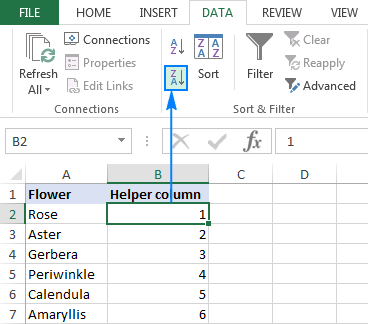As shown in the screenshot below, this will sort not only the numbers in column B, but also the original items in column A, reversing the order of rows: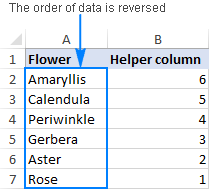Now you can safely delete the helper column since you do not need it any longer.

Tip: How to quickly fill a column with serial numbers

The fastest way to populate a column with a sequence of numbers is by using the Excel AutoFill feature:

• Type 1 into the first cell and 2 into the second cell (cells B2 and B3 in the screenshot below).
• Select the cells where you've just entered the numbers and double-click the lower right corner of the selection.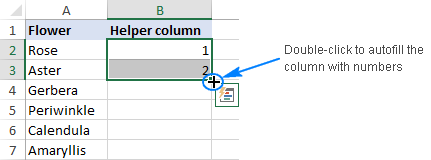That's it! Excel will autofill the column with serial numbers up to the last cell with data in the adjacent column.

### How to flip a table in Excel

The above method also works for reversing the data order in multiple columns: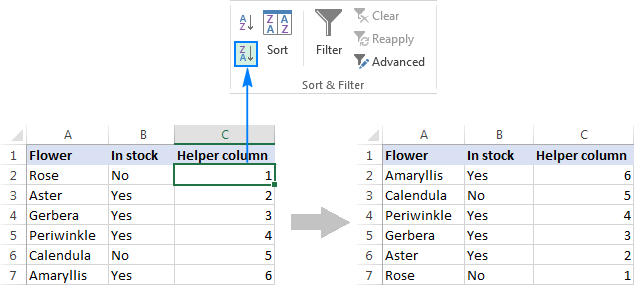Sometimes (most often when you select the whole column of numbers prior to sorting) Excel might display the Sort Warning dialog. In this case, check the Expand the selection option, and then click the Sort button.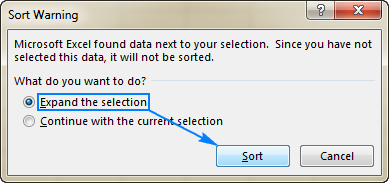Tip. If you'd like to rotate data from rows to columns or vice versa, use the Excel Transpose feature or other ways to convert rows to columns demonstrated in How to transpose in Excel.

### How to flip columns in Excel using a formula

Another way to flip a column upside down is by using this generic formula:

INDEX(range, ROWS(range))

For our sample data set, the formula goes as follows:

`=INDEX(\$A\$2:\$A\$7,ROWS(A2:\$A\$7))`

…and reverses column A impeccably: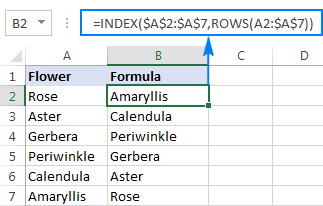#### How this formula works

At the heart of the formula is the INDEX(array, row_num, [column_num]) function, which returns the value of an element in array based on the row and/or column numbers you specify.

In the array, you feed the entire list you want to flip (A2:A7 in this example).

The row number is worked out by the ROWS function. In its simplest form, ROWS(array) returns the number of rows in array. In our formula, it's the clever use of the relative and absolute references that does the "flip column" trick:

• For the first cell (B2), ROWS(A2:\$A\$7) returns 6, so INDEX gets the last item in the list (the 6th item).
• In the second cell (B3), the relative reference A2 changes to A3, consequently ROWS(A3:\$A\$7) returns 5, forcing INDEX to fetch the second to last item.

In other words, ROWS creates a kind of decrementing counter for INDEX so that it moves from the last item toward the first item.

Tip: How to replace formulas with values

Now that you have two columns of data, you may want to replace formulas with calculated values, and then delete an extra column. For this, copy the formula cells, select the cells where you'd like to paste the values, and press Shift+F10 then V, which is the fastest way to apply Excel's Paste Special > Values option.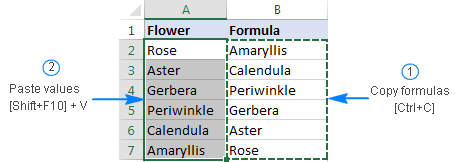### How to flip columns in Excel with VBA

If you have some experience with VBA, you can use the following macro to reverse the data order vertically in one or several columns:

```Sub FlipColumns()
Dim Rng As Range
Dim WorkRng As Range
Dim Arr As Variant
Dim i As Integer, j As Integer, k As Integer

On Error Resume Next

xTitleId = "Flip columns vertically"
Set WorkRng = Application.Selection
Set WorkRng = Application.InputBox("Range", xTitleId, WorkRng.Address, Type:=8)
Arr = WorkRng.Formula
Application.ScreenUpdating = False
Application.Calculation = xlCalculationManual

For j = 1 To UBound(Arr, 2)
k = UBound(Arr, 1)
For i = 1 To UBound(Arr, 1) / 2
xTemp = Arr(i, j)
Arr(i, j) = Arr(k, j)
Arr(k, j) = xTemp
k = k - 1
Next
Next

WorkRng.Formula = Arr
Application.ScreenUpdating = True
Application.Calculation = xlCalculationAutomatic

End Sub
```

#### How to use the Flip Columns macro

1. Open the Microsoft Visual Basic for Applications window (Alt + F11).
2. Click Insert > Module, and paste the above code in the Code window.
3. Run the macro (F5).
4. The Flip Columns dialog pops up prompting you to select a range to flip: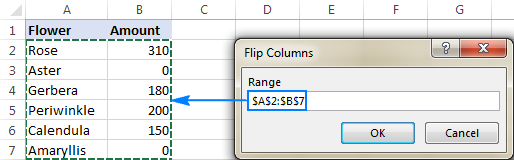You select one or more columns using the mouse, not including the column headers, click OK and get the result in a moment.

To save the macro, be sure to save your file as an Excel macro-enabled workbook.

### How to flip data in Excel preserving formatting and formulas

With the above methods, you can easily reverse the data order in a column or table. But what if you not only wish to flip values, but cell formats too? Additionally, what if some data in your table is formula-driven, and you want to prevent formulas from being broken when flipping cells in columns? In this case, you can use the Flip feature included with our Ultimate Suite for Excel.

Supposing you have a nicely formatted table like shown below, where some columns contain values and some columns have formulas: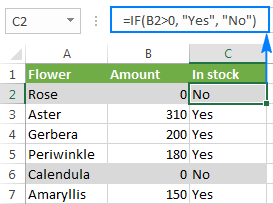You are looking to flip the columns in your table keeping both formatting (grey shading for rows with zero amount) and correctly calculated formulas. This can be done in two quick steps:

1. With any cell in your table selected, go to the Ablebits Data tab > Transform group, and click Flip > Vertical Flip.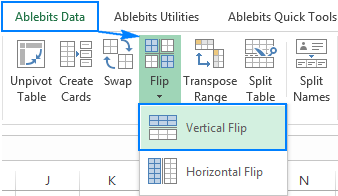2. In the Vertical Flip dialog window, configure the following options:
• In the Select your range box, check the range reference and make sure the header row is not included.
• Select the Adjust cell references option and check the Preserve formatting box.
• Optionally, choose to Create a back up copy (selected by default).
• Click the Flip button.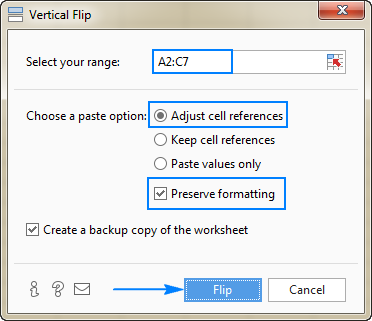Done! The order of data in the table is reversed, the formatting is kept, and cell references in the formulas are appropriately adjusted: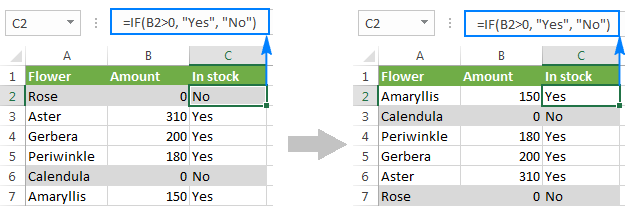## Flip data in Excel horizontally

So far in this tutorial, we have flipped columns upside down. Now, let's look at how to reverse data order horizontally, i.e. flip a table from left to right.

### Reverse data order horizontally with VBA

Here is a simple macro that can quickly flip data in your Excel table horizontally:

```Sub FlipDataHorizontally()

Dim Rng As Range
Dim WorkRng As Range
Dim Arr As Variant
Dim i As Integer, j As Integer, k As Integer

On Error Resume Next

xTitleId = "Flip Data Horizontally"
Set WorkRng = Application.Selection
Set WorkRng = Application.InputBox("Range", xTitleId, WorkRng.Address, Type:=8)
Arr = WorkRng.Formula

Application.ScreenUpdating = False
Application.Calculation = xlCalculationManual

For i = 1 To UBound(Arr, 1)
k = UBound(Arr, 2)
For j = 1 To UBound(Arr, 2) / 2
xTemp = Arr(i, j)
Arr(i, j) = Arr(i, k)
Arr(i, k) = xTemp
k = k - 1
Next
Next

WorkRng.Formula = Arr
Application.ScreenUpdating = True
Application.Calculation = xlCalculationAutomatic
End Sub
```

To add the macro to your Excel workbook, please follow these steps. As soon as you run the macro, the following dialog window will show up, asking you to select a range: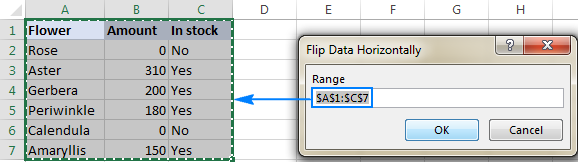You select the entire table, including the header row, and click OK. In a moment, the data order in rows in reversed: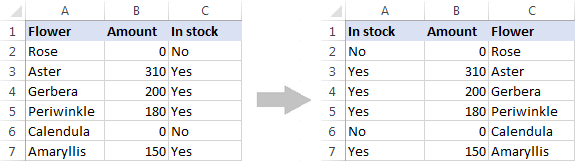### Flip data in rows with Ultimate Suite for Excel

Similarly to flipping columns, you can use our Ultimate Suite for Excel to reverse the order data in rows. Just select a range of cells you want to flip, go to the Ablebits Data tab > Transform group, and click Flip > Horizontal Flip.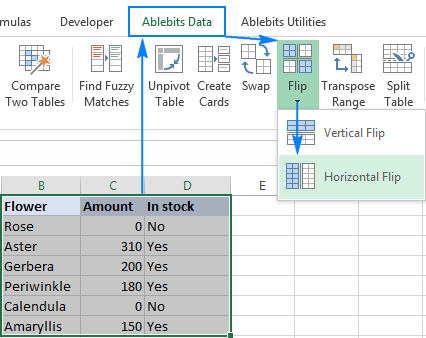In the Horizontal Flip dialog window, choose the options appropriate for your data set. In this example, we are working with values, so we choose Paste values only and Preserve Formatting: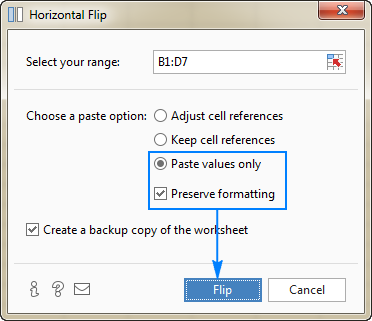Click the Flip button, and your table will be reversed from left to right in the blink of an eye.

This is how you flip data in Excel. I thank you for reading and hope to see you on our blog next week!

### 15 responses to "How to flip data in Excel: reverse columns vertically and rows horizontally"

1.Charly Paredes says:

Thanks, for the article.

2.yuvraj bhalke says:

we need this details

3.Marvan says:

Thank you so much. This is a life saver! I being searching for a solution like this nearly for an hour now. Thank you again!

4.Roman says:

To flip horizontally, you can also simply transpose first, then sort A-Z (if necessary add column with numbers first to use to), then transpose back. Done.

•max says:

excelent solution

5.Edward says:

Thanks Roman, that was an extremely easy way to flip horizontally, took seconds.

6.Ishan says:

Thanks.

7.juan figueroa says:

hello and thanks,
have you the function version of both pls?
thanks again!

8.Glyn Tomkins says:

good article - might i offer another elegant, quick way to flip the rows ie horizontal flip?

Transpose the data (copy - paste special - transpose)
use your method for flipping columns - i.e. the numbers and a sort

Transpose the data back again

9.Derek says:

Thank you for the Index(range, Rows(range)) trick! Worked perfectly.

10.M A Motin Sobuj says:

Thanks a lot for this nice post

11.DMarie says:

Excellent macro for horizontal flip - THANK YOU!

12.MevaD says:

Thanks

13.Simon says:

Many, many thanks. This tutorial has saved me a lot of faffing and time. Don't think I would have worked this out without some help as the method is not what you would call intuitive. Nonetheless, it's very easy when you know how. Now I do to. Cheers.

14.Gabi says:

Hello,
Thank you so much!